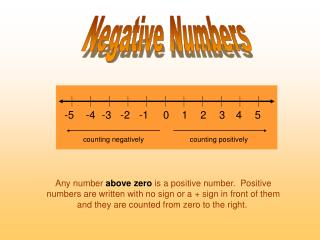DownloadDownload PresentationNegative Numbers

# Negative Numbers

Download Presentation## Negative Numbers

- - - - - - - - - - - - - - - - - - - - - - - - - - - E N D - - - - - - - - - - - - - - - - - - - - - - - - - - -
##### Presentation Transcript

1. Negative Numbers -5 -4 -3 -2 -1 0 1 2 3 4 5 counting negatively counting positively Any number above zero is a positive number. Positive numbers are written with no sign or a + sign in front of them and they are counted from zero to the right.

2. -5 -4 -3 -2 -1 0 1 2 3 4 5 counting negatively counting positively Any number below zero is a negative number. Negative numbers are written with a - sign in front of them and they are counted from zero to the left. Always look at the sign in front of a number to see if it is positive or negative.

3. -5 -4 -3 -2 -1 0 1 2 3 4 5 counting negatively counting positively Remember: Positive numbers get bigger the further we move right, so 5 is bigger than 2. Negative numbers get smaller the further we move left, so -5 is smaller than -2.

4. -5 -4 -3 -2 -1 0 1 2 3 4 5 counting negatively counting positively Example: Which word, “bigger” or “smaller”, would fit correctly In each of these gaps? smaller -7 is ___________than -1 +62 is ___________than -71 -136 is ___________than -36 bigger smaller

5. Why do negative numbers "get bigger"? As you extend a number line showing negative numbers,they seem to get “bigger.” -10 -9 -8 -7 -6 -5 -4 -3 -2 -1 0 1 2

6. -10 -9 -8 -7 -6 -5 -4 -3 -2 -1 0 1 2 The numbers seem to increase in value as they go down the number line. But as the negative number gets bigger, the value gets lower. -10 is a larger number than -5, so it is further below zero. If you look at the number line you can see that -10 is less than -5.

7. a d m s w e -4 -2 0 2 4 Let's Practice -10 8 What value is letter w? What value is letter a? Which value is bigger -10 or 8? Even though 10 is a bigger number than 8, 8 is bigger because 10 is negative.

8. o Spring 7 C o o Fall -4 C Winter -12 C If it helps you remember, think about the weather. As temperature gets lower the negative numbers seem to get bigger.

9. Temperature increase and decrease When the weather changes the temperature can go up or down. If a temperature goes up, gets warmer, it is a temperature increase. If a temperature goes down, gets colder, it is a temperature decrease.

10. During the summer when you wake up, the morning temperature could be 3 C. By lunchtime it is up to 10 C. o o This is a temperature increase of 7 C. 15 15 10 10 5 5 o 0 0 -5 -5 Example:

11. In the early afternoon the temperature is 15 C. By early evening the temperature goes down to 12 C. o o 15 This is a temperature decrease of 3 C. 15 10 10 5 5 0 o 0 -5 -5

12. During winter the early morning temperature could be -3 C. By lunch it is up to 6 C. o o o o o From -3 C to 0 C is 3 C. 15 15 10 10 This is a temperature increase of 9 C. 5 5 o 0 0 -5 -5 o o o From 0 C to 6 C is a further 6 C. o o The total of 3 C + 6 C makes 9 C. o But what happens if we have negative temperatures? Example:

13. In the early afternoon the temperature is 3 C. By early evening the temperature goes down to -5 C. o o 15 o o o From 3 C to 0 C is 3 C. 15 10 10 5 0 5 0 -5 This is a temperature decrease of 8 C. -5 o o o o The total of 3 C + 5 C makes 8 C. o o o From 0 C to -5 C is a further 5 C.

14. 14 10 o 2 C 6 o C Let's Practice What temperature is shown on the thermometer?

15. 35 25 o -5 C 10 0 o C What temperature is shown on the thermometer?